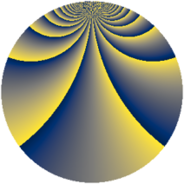# Properties

 Label 1600.2.byLevel $1600$ Weight $2$ Character orbit 1600.by Rep. character $\chi_{1600}(63,\cdot)$ Character field $\Q(\zeta_{20})$ Dimension $464$ Sturm bound $480$

# Related objects

## Defining parameters

 Level: $$N$$ $$=$$ $$1600 = 2^{6} \cdot 5^{2}$$ Weight: $$k$$ $$=$$ $$2$$ Character orbit: $$[\chi]$$ $$=$$ 1600.by (of order $$20$$ and degree $$8$$) Character conductor: $$\operatorname{cond}(\chi)$$ $$=$$ $$100$$ Character field: $$\Q(\zeta_{20})$$ Sturm bound: $$480$$

## Dimensions

The following table gives the dimensions of various subspaces of $$M_{2}(1600, [\chi])$$.

Total New Old
Modular forms 2016 496 1520
Cusp forms 1824 464 1360
Eisenstein series 192 32 160

## Trace form

 $$464q + 16q^{5} - 20q^{9} + O(q^{10})$$ $$464q + 16q^{5} - 20q^{9} + 16q^{13} - 8q^{17} + 12q^{21} - 8q^{25} + 20q^{29} - 28q^{33} + 16q^{37} - 12q^{41} + 36q^{45} - 32q^{53} - 4q^{57} + 12q^{61} - 24q^{65} + 20q^{69} - 8q^{73} + 92q^{77} + 72q^{81} + 64q^{85} + 20q^{89} + 76q^{93} - 152q^{97} + O(q^{100})$$

## Decomposition of $$S_{2}^{\mathrm{new}}(1600, [\chi])$$ into newform subspaces

The newforms in this space have not yet been added to the LMFDB.

## Decomposition of $$S_{2}^{\mathrm{old}}(1600, [\chi])$$ into lower level spaces

$$S_{2}^{\mathrm{old}}(1600, [\chi]) \cong$$ $$S_{2}^{\mathrm{new}}(100, [\chi])$$$$^{\oplus 5}$$$$\oplus$$$$S_{2}^{\mathrm{new}}(400, [\chi])$$$$^{\oplus 3}$$$$\oplus$$$$S_{2}^{\mathrm{new}}(800, [\chi])$$$$^{\oplus 2}$$